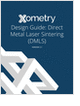Formula HomeMechanics of MaterialsStressStrainHooke's LawApplicationsPressure VesselsRosette Strain GagesFailure CriteriaCalculatorsStress TransformStrain TransformPrincipal StressPrincipal StrainElastic ConstantsResourcesBibliography

Learn the best principles to negotiate the salary you deserve!

Essentials of Manufacturing

Information, coverage of important developments and expert commentary in manufacturing.

Metal 3D Printing Design Guide

Direct Metal Laser Sintering (DMLS) 3D printing for parts with reduced cost and little waste.

CNC Machining Design Guide

more free magazinesStrain Rosette for Strain MeasurementA wire strain gage can effectively measure strain in only one direction. To determine the three independent components of plane strain, three linearly independent strain measures are needed, i.e., three strain gages positioned in a rosette-like layout.Consider a strain rosette attached on the surface with an angle a from the x-axis. The rosette itself contains three strain gages with the internal angles b and g, as illustrated on the right.

Suppose that the strain measured from these three strain gages are ea, eb, and ec, respectively.

The following coordinate transformation equation is used to convert the longitudinal strain from each strain gage into strain expressed in the x-y coordinates,Applying this equation to each of the three strain gages results in the following system of equations,These equations are then used to solve for the three unknowns, ex, ey, and exy.

 Note: 1 The above formulas use the strain measure exy as opposed to the engineering shear strain gxy,. To use gxy, the above equations should be adjusted accordingly. 2 The free surface on which the strain rosette is attached is actually in a state of plane stress, while the formulas used above are for plane strain. However, the normal direction of the free surface is indeed a principal axis for strain. Therefore, the strain transform in the free surface plane can be applied.
Special Cases of Strain Rosette LayoutsCase 1: 45º strain rosette aligned with the x-y axes, i.e., a = 0º, b = g = 45º.Case 2: 60º strain rosette, the middle of which is aligned with the y-axis, i.e., a = 30º, b = g = 60º.Learn the best principles to negotiate the salary you deserve!Essentials of Manufacturing

Information, coverage of important developments and expert commentary in manufacturing.Metal 3D Printing Design Guide

Direct Metal Laser Sintering (DMLS) 3D printing for parts with reduced cost and little waste.CNC Machining Design Guide